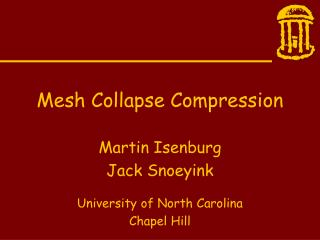Download PresentationMesh Collapse CompressionMesh Collapse Compression - PowerPoint PPT Presentation

Download PresentationMesh Collapse Compression
An Image/Link below is provided (as is) to download presentation

Download Policy: Content on the Website is provided to you AS IS for your information and personal use and may not be sold / licensed / shared on other websites without getting consent from its author. While downloading, if for some reason you are not able to download a presentation, the publisher may have deleted the file from their server.

- - - - - - - - - - - - - - - - - - - - - - - - - - - E N D - - - - - - - - - - - - - - - - - - - - - - - - - - -
Presentation Transcript

1. Mesh Collapse Compression Martin Isenburg Jack Snoeyink University of North Carolina Chapel Hill

2. Introduction A novel scheme for encoding triangle mesh topology.

3. Introduction A novel scheme for encoding triangle mesh topology. • triangle mesh

4. Introduction A novel scheme for encoding triangle mesh topology. • triangle mesh • encoding

5. Introduction A novel scheme for encoding triangle mesh topology. • triangle mesh • encoding • topology

6. Triangle Mesh • Computer Graphics • Surface description • Hardware support • Used everyday • Used everywhere • Used by everybody • Increasingly complex

7. Encoding • Compressed representation • Decrease storage and transmission time 011101101011...

8. Topology geometry versus topology x0 y0 z0 0 1 2 x1 y1 z1 2 3 0 x2 y2 z2 2 3 1 x3 y3 z3 2 4 3 x4 y4 z4 5 4 3 . . . . . xn yn zn . . . . . . . . . . . . . . .

9. Overview • previous work • simple meshes • video • example • general meshes • results • summary

10. Previous work • Deering • Keeler Westbrook • Taubin Rossignac • Tauma Gotsman • Rossignac • DeFloriani et al • Isenburg Snoeyink

11. Previous work Geometry Compression • Deering • Keeler Westbrook • Taubin Rossignac • Tauma Gotsman • Rossignac • DeFloriani et al • Isenburg Snoeyink

12. Previous work Short Encodings of Planar Graphs and Maps 4.6 bpv (4.6) • Deering • Keeler Westbrook • Taubin Rossignac • Tauma Gotsman • Rossignac • DeFloriani et al • Isenburg Snoeyink

13. Previous work Topological Surgery 2.2 ~ 4.8 bpv (--) • Deering • Keeler Westbrook • Taubin Rossignac • Tauma Gotsman • Rossignac • DeFloriani et al • Isenburg Snoeyink

14. Previous work Triangle Mesh Compression 0.2 ~ 2.9 bpv (--) • Deering • Keeler Westbrook • Taubin Rossignac • Tauma Gotsman • Rossignac • DeFloriani et al • Isenburg Snoeyink

15. Previous work Edgebreaker 3.4 ~ 4.0 bpv (4.0) • Deering • Keeler Westbrook • Taubin Rossignac • Tauma Gotsman • Rossignac • DeFloriani et al • Isenburg Snoeyink

16. Previous work A Simple and Efficient Encoding for Triangle Meshes 4.2 ~ 5.4 bpv (6.0) • Deering • Keeler Westbrook • Taubin Rossignac • Tauma Gotsman • Rossignac • DeFloriani et al • Isenburg Snoeyink

17. Previous work Face Fixer 3.9 ~ 4.1 bpv (6.0) • Deering • Keeler Westbrook • Taubin Rossignac • Tauma Gotsman • Rossignac • DeFloriani et al • Isenburg Snoeyink

18. Simple Meshes (1) the mesh is a surface composed of triangles (2) the mesh has no boundary (3) the mesh has no holes (4) the mesh has no handles

19. Simple Mesh

20. Mesh Collapse Compression i Input: Output: a simple mesh - a sequence of code words - a permutation of vertices

21. Compression Scheme (1) initialize - declare arbitrary directed mesh edge as current edge

22. Compression Scheme (2) loop until done (and usually): - contract current edge - record the removed vertex - record the degree - select new current edge

23. Compression Scheme (2) loop until done (but occasionally): - divide mesh along current edge - record start symbol - continue on one mesh part - record end symbol - continue on other mesh part

24. Digons A triangulation withexception of theouter face that isbound by only twoedges.

25. From Mesh to Digon mc-edge new mc-edge mc-vertex cut and open rearrange

26. Various Digons dividing edge simple simple simple trivial complex

27. Encode Algorithm encode( Mesh mesh, Codec codec ) { stack.push( digonify( mesh ) ); while ( not stack.empty( ) ) { digon = stack.pop( ); while ( not digon.trivial ( ) ) { if ( digon.complex() ) { subdigon = mc-divide( digon ); stack.push( subdigon ); codec.pushCode( “S” ); } else { degree = mc-contract( digon ); codec.pushCode( degree ); } } codec.push( “E” ); } }

28. mc-contract mc-edge loop new mc-edge contract contract remove select

29. mc-divide dividing edge mc-edge mc-edge divide select

30. Video

31. Example

32. Example

33. Example mc-vertex

34. Example mc-edge

35. Example mc-edge digonify

36. Example

37. Example removed vertex degree of removed vertex mc-contract

38. Example

39. Example removed vertex degree of removed vertex mc-contract

40. Example

41. Example removed vertex degree of removed vertex mc-contract

42. Example

43. Example removed vertex degree of removed vertex mc-contract

44. Example

45. Example divided vertex mc-divide

46. Example divided vertex mc-divide

47. Example push on stack

48. Example

49. Example degree of removed vertex mc-contract

50. Example